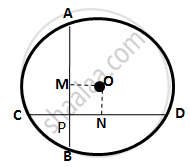Share

Ab and Cd Are Two Equal Chords of a Circle with Centre O Which Intersect Each Other at Right Angle at Point P. If Om⊥ Ab and On ⊥ Cd; Show that Ompn is a Square. - ICSE Class 10 - Mathematics

ConceptChord Properties - Chords Equidistant from the Center Are Equal (Without Proof)

Question

AB and CD are two equal chords of a circle with centre O which intersect each other at right
angle at point P. If  OM⊥ AB and ON ⊥ CD; show that OMPN is a square.

SolutionClearly, all the angles of OMPN are 90°
OM⊥ AB and ON ⊥CD
∴ BM =1/2 = AB=1/2  CD = CN  ………….. (i)
(Perpendicular drawn from the centre of a circle to a chord bisects it)
As the two equal chords AB and CD intersect at point P inside
The circle,

∴ AP = DP  and CP = BP         ……………. (ii)
Now, CN - CP =  BM - BP (by (i) and (ii))
⇒ PN MP
∴ Quadrilateral OMPN is a square

Is there an error in this question or solution?

Video TutorialsVIEW ALL 

Solution Ab and Cd Are Two Equal Chords of a Circle with Centre O Which Intersect Each Other at Right Angle at Point P. If Om⊥ Ab and On ⊥ Cd; Show that Ompn is a Square. Concept: Chord Properties - Chords Equidistant from the Center Are Equal (Without Proof).
S